# Device couplings - TLM

This section describes Transmission line model device coupling, including Single Phase Device Coupling component, Three Phase Device Coupling component and Four Phase Device Coupling component.

TLM device coupling components are used to partition the complete power electronics circuit into separate circuits that are emulated on different HIL devices. These components are only used in multi-HIL configurations where HIL devices are paralleled. Device coupling components define the communication variables between HIL devices, that are exchanged true High Speed Serial Link (HSSL). This block introduces the variable time delay (~2 µs) between measured variables and corresponding controlled sources, which is negligible for most practical systems. This delay depends on the amount of data that has to be transmitted true HSSL.

TLM technique models discrete reactive components as transmission-line sections known as stubs, each of which has its own inductance and capacitance. TLM stubs are one port devices; The inductor can be represented as short circuit stub, while the capacitor can be represented as open circuit stub. Every reactive component in the circuit can be replaced by TLM stub. Meanwhile, TLM links are two port-devices. TLM link also has its own capacitance and inductance. If capacitance is dominant, the link can be considered as capacitive. In that case, the characteristic impedance of the capacitive link can be written as Zlk=L/T. If inductance is dominant, the link can be considered as inductive and corresponding characteristic impedance can be written as Zlk=T/C. In the previous equations, T represents simulation step duration.

TLM coupling component implementation is based on TLM links. The main advantage of TLM couplings, compared to the ideal transformer based coupling components, is that TLM couplings are symmetrical components. Both sides of the TLM couplings are voltage sources behind an impedance. Because of this property, TLM coupling rotation is not important and they will not introduce any topological conflict in the circuit. Figure Figure 1 shows structure of the TLM coupling component.

There are three TLM device coupling in the library: single-phase, three-phase and four-phase device couplings.

For more details about coupling placement please refer to Coupling component placement and parametrization - TLM based couplings.

## Single Phase TLM Device Coupling

A Single Phase TLM (Transmission Line Model) Device Coupling component is based on a lossless transmission line model.

There are five parameters available: Coupling Type, values for L or C, Embedded inductors/capacitors, TLM / Embedded components ratio, and Ratio. Coupling type can be either inductive or capacitive. According to the selected type a parameter for inductor value or capacitor value is available. TLM coupling behaves as an additional inductor in the circuit or as an additional capacitor. If Embedded inductors/capacitors is enabled embedded inductors/capacitors will be added to both sides of the coupling, as it is shown in Figure 2 and Figure 3.

If embedded components are added, it is necessary to specify how the impedance will be divided between TLM coupling and embedded components. That can be done using the TLM / Embedded components ratio and Ratio properties. If the 'Automatic' option is selected, the ratio will be determined by the discretization method. If the 'Manual' option is selected, the ratio can be explicitly set to meet the model's requirements. It must be in range (0,1). Best results for most practical use cases are obtained if Ratio is in range (0.001, 0.01).

An existing inductor or capacitor can be replaced with a corresponding TLM coupling. In this case, the TLM coupling inductance/capacitance needs to be the same as inductance/capacitance of the replaced element. If Embedded inductors/capacitors is enabled, inductance/capacitance will be divided between TLM and embedded components. In that case, the best results are obtained. It is recommended to use TLM couplings in this way. Check Coupling component placement and parametrization - TLM based couplings for usage examples.

When a TLM coupling is used, the simulation step cannot be set to the Auto option and therefore must be defined explicitly.

The main advantage of TLM couplings, compared to the ideal transformer based coupling components, is that TLM couplings are symmetrical components. Both sides of the TLM couplings are voltage sources behind an impedance. Because of this property, TLM coupling rotation is not important and they will not introduce any topological conflict in the circuit. The main disadvantage is that they will add some additional inductance or capacitance to the circuit. However, it is better to replace an existing inductor/capacitor with a TLM coupling in order to get better results. For more details about TLM coupling placement please refer to Coupling component placement and parametrization - TLM based couplings

Table 1. Single Phase TLM Device Coupling component in the Schematic Editor core library
component component dialog window component parametersSingle Phase TLM Device Coupling• Coupling Type - Inductive or Capacitive
• L/C - inductance or capacitance value
• Embedded inductors/capacitors
• TLM/Embedded components ratio - Automatic or Manual
• Ratio - Must be in range (0,1)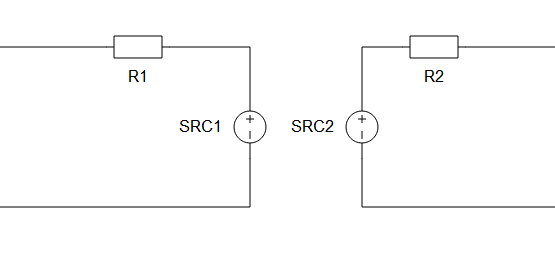Figure 1. The Schematic block diagram of a Single Phase TLM Device Coupling component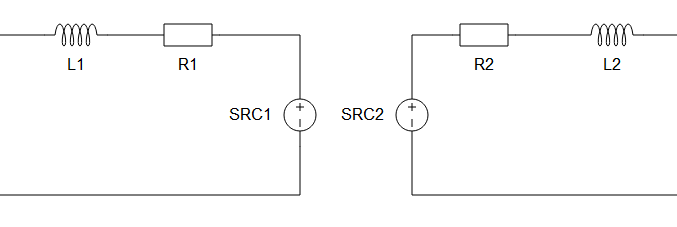Figure 2. The Schematic block diagram of a Single Phase TLM Coupling component with embedded inductors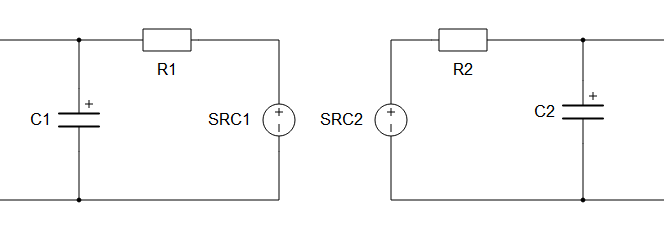Figure 3. The Schematic block diagram of a Single Phase TLM Device Coupling component with embedded capacitors

## Three Phase TLM Device Coupling component

A Three Phase TLM (Transmission Line Model) Device Coupling component is based on a Single Phase TLM Device Coupling component.

There are five parameters available: Coupling Type, values for L or C, Embedded inductors/capacitors, TLM / Embedded components ratio, and Ratio. Coupling type can be either inductive or capacitive. According to the selected type a parameter for inductor value or capacitor value is available. TLM coupling behaves as an additional inductor in the circuit or as an additional capacitor. If Embedded inductors/capacitors is enabled embedded inductors/capacitors will be added to both sides of the coupling, as it is shown in Figure 2 and Figure 3.

If embedded components are added, it is necessary to specify how the impedance will be divided between TLM coupling and embedded components. That can be done using the TLM / Embedded components ratio and Ratio properties. If the 'Automatic' option is selected, the ratio will be determined by the discretization method. If the 'Manual' option is selected, the ratio can be explicitly set to meet the model's requirements. It must be in range (0,1). Best results for most practical use cases are obtained if Ratio is in range (0.001, 0.01).

An existing inductor or capacitor can be replaced with a corresponding TLM coupling. In this case, the TLM coupling inductance/capacitance needs to be the same as inductance/capacitance of the replaced element. If Embedded inductors/capacitors is enabled, inductance/capacitance will be divided between TLM and embedded components. In that case, the best results are obtained. It is recommended to use TLM couplings in this way. Check Coupling component placement and parametrization - TLM based couplings for usage examples.

When a TLM coupling is used, the simulation step cannot be set to the Auto option and therefore must be defined explicitly.

The bilinear discretization method is recommended since TLM coupling is based on this approach. But, if the TLM inductance(capacitance) is relatively small, trapezoidal discretization can be used. TLM coupling is in general more robust than the Ideal Transformer coupling. TLM coupling in combination with bilinear or trapezoidal discretization method guaranties stability in the most of practical use cases, so there is no need for an additional stability analysis.

Table 2. Three Phase TLM Device Coupling component in the Schematic Editor core library
component component dialog window component parameters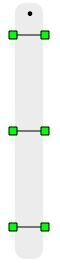Three Phase TLM Device Coupling• Coupling Type - Inductive or Capacitive
• L/C - inductance or capacitance value
• Embedded inductors/capacitors
• TLM/Embedded components ratio - Automatic or Manual
• Ratio - Must be in range (0,1)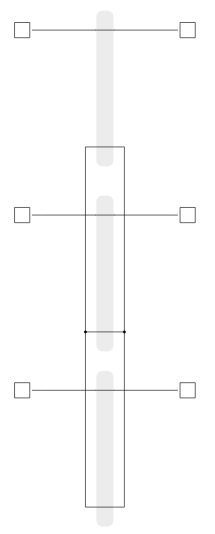Figure 4. The schematic block diagram of a Three Phase TLM Device Coupling component

## Four Phase TLM Device Coupling component

A Four Phase TLM (Transmission Line Model) Device Coupling component is based on a Single Phase TLM Device Coupling component.

There are five parameters available: Coupling Type, values for L or C, Embedded inductors/capacitors, TLM / Embedded components ratio, and Ratio. Coupling type can be either inductive or capacitive. According to the selected type a parameter for inductor value or capacitor value is available. TLM coupling behaves as an additional inductor in the circuit or as an additional capacitor. If Embedded inductors/capacitors is enabled embedded inductors/capacitors will be added to both sides of the coupling, as it is shown in Figure 2 and Figure 3.

If embedded components are added, it is necessary to specify how the impedance will be divided between TLM coupling and embedded components. That can be done using the TLM / Embedded components ratio and Ratio properties. If the 'Automatic' option is selected, the ratio will be determined by the discretization method. If the 'Manual' option is selected, the ratio can be explicitly set to meet the model's requirements. It must be in range (0,1). Best results for most practical use cases are obtained if Ratio is in range (0.001, 0.01).

An existing inductor or capacitor can be replaced with a corresponding TLM coupling. In this case, the TLM coupling inductance/capacitance needs to be the same as inductance/capacitance of the replaced element. If Embedded inductors/capacitors is enabled, inductance/capacitance will be divided between TLM and embedded components. In that case, the best results are obtained. It is recommended to use TLM couplings in this way. Check Coupling component placement and parametrization - TLM based couplings for usage examples.

When a TLM coupling is used, the simulation step cannot be set to the Auto option and therefore must be defined explicitly.

The main advantage of TLM couplings, compared to the ideal transformer based coupling components, is that TLM couplings are symmetrical components. Both sides of the TLM couplings are voltage sources behind an impedance. Because of this property, TLM coupling rotation is not important and they will not introduce any topological conflict in the circuit. The main disadvantage is that they will add some additional inductance or capacitance to the circuit. However, it is better to replace an existing inductor/capacitor with a TLM coupling in order to get better results. For more details about TLM coupling placement please refer to Coupling component placement and parametrization - TLM based couplings

Table 3. Four Phase TLM Device Coupling component in the Schematic Editor core library
component component dialog window component parameters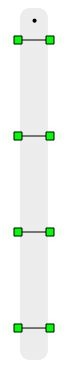Four Phase TLM Device Coupling• Coupling Type - Inductive or Capacitive
• L/C - inductance or capacitance value
• Embedded inductors/capacitors
• TLM/Embedded components ratio - Automatic or Manual
• Ratio - Must be in range (0,1)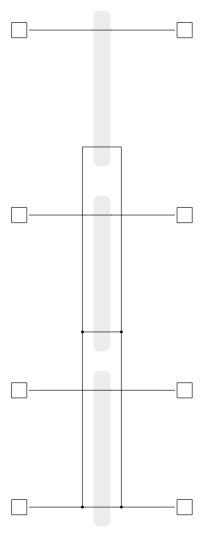Figure 5. The schematic block diagram of a Four Phase TLM Device Coupling component